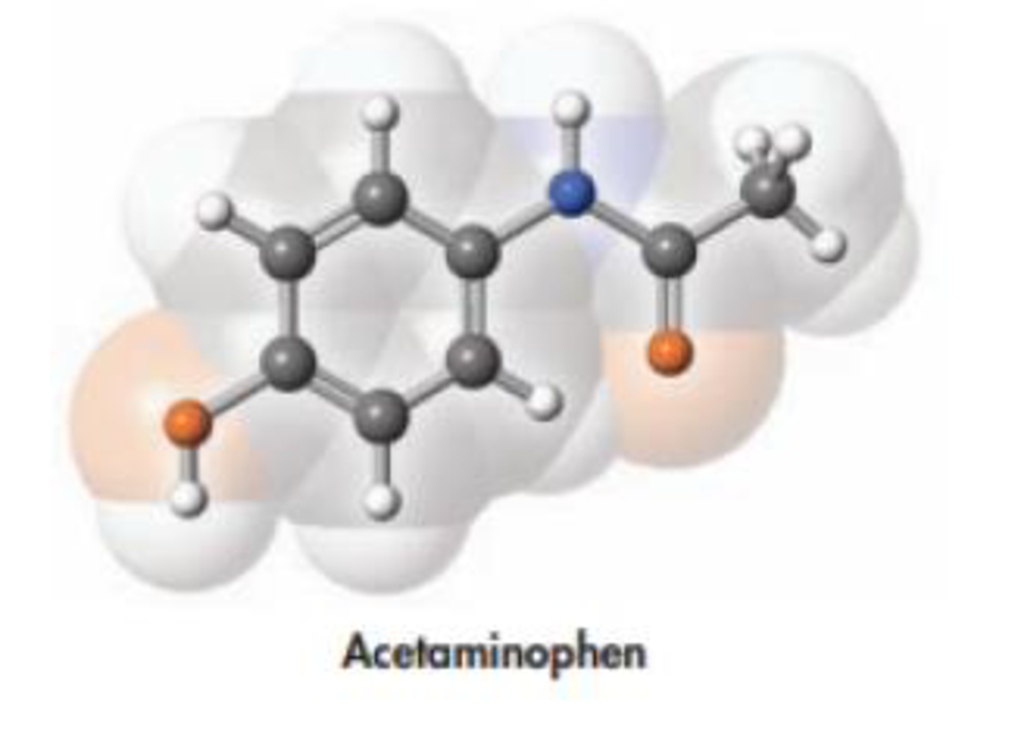Chapter 2, Problem 77PS

Chapter
Section
Textbook Problem

Acetaminophen, whose structure is drawn below, is the active ingredient in some nonprescription pain killers. The recommended dose for an adult is two 500-mg caplets. How many molecules make up one dose of this drug?Interpretation Introduction

Interpretation: The number of molecules present in two 500mg  caplets is to be calculated.

Concept introduction:

• Molar mass of a molecule can be calculated from its molecular formula by taking the sum of atomic masses of all the elements present in it.
• Conversion formula for mass of a molecule into number moles,

Numberofmoles=MassingramsMolarmass

• Equation for finding number of molecules from number of moles of a molecule,

Numberofmolecule=numberofmolesofmolecule×6.022×1023molecules/mol

Explanation

The amount of two 500mg acetaminophen caplets is 1000mg.

The molar mass of acetaminophen is 151.163g.

Conversion formula for mass of a molecule into number moles,

Numberofmoles=MassingramsMolarmass

Therefore,

The number moles of acetaminophen is,

Numberofmoles=1000g151.165g/mol=6

Still sussing out bartleby?

Check out a sample textbook solution.

See a sample solution

The Solution to Your Study Problems

Bartleby provides explanations to thousands of textbook problems written by our experts, many with advanced degrees!

Get Started

Find more solutions based on key concepts